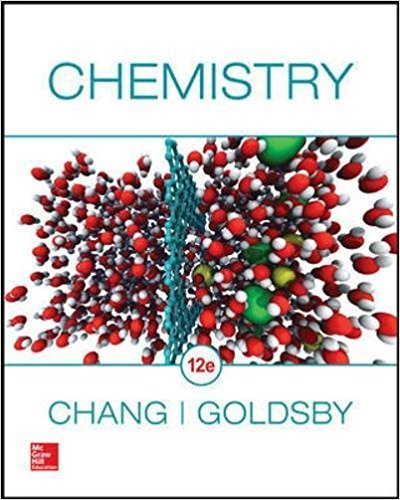×
Get Full Access to Chemistry - 12 Edition - Chapter 2 - Problem 2.63
Get Full Access to Chemistry - 12 Edition - Chapter 2 - Problem 2.63

×

# Which pair of species has the same number of electrons?ISBN: 9780078021510 98

## Solution for problem 2.63 Chapter 2

Chemistry | 12th Edition

• Textbook Solutions
• 2901 Step-by-step solutions solved by professors and subject experts
• Get 24/7 help from StudySoup virtual teaching assistantsChemistry | 12th Edition

4 5 1 351 Reviews
23
5
Problem 2.63

Pair the following species that contain the same number of electrons: $$\mathrm{Ar,}\mathrm{\ Sn}^{4+},\ \mathrm{F}^-,\mathrm{\ Fe}^{3+},\mathrm{\ P}^{3-},\mathrm{\ V}\mathrm{,\ Ag}^+,\mathrm{\ N}^{3-}$$

Step-by-Step Solution:

Step 1 of 2

Take the number of electrons based on the neutral element and add one for each negative charge or subtract one for each positive charge to get the number of electrons for each species.

Step 2 of 2

## Discover and learn what students are asking

Unlock Textbook Solution

Enter your email below to unlock your verified solution to:

Solved: Pair the following species that contain the same